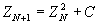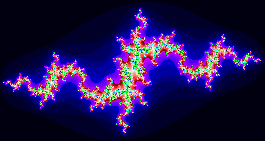Columns

Beginners

Synthesis

Processing

Real-Time

Features

AXCsound

Composing

Ecological Modeling

Departments

Editorial

Contribute

Back Issues

## Sound Generation with the Julia Set

Hans Mikelson

hljmm@werewolf.net

Introduction

Fractals can be used to create beautiful and interesting images. You have probably seen the beautiful images of the Mandelbrot set and may have seen images of its cousin the Julia set. A typical Csounder viewing a fractal will ask "It looks nice but how does it sound?" In this article I present two different ways to convert fractal images to sound.

The Julia Set

The Julia set is based on the iteration of the formulawhere Z and C are complex numbers. Z is initialized to a coordinate in the complex plane and C is initialized to some constant value. This is different from the Mandelbrot set where Z is initialized to zero and C is initialized to a coordinate. Typically a rectangular grid is scanned and the number of iterations it takes for |Z| to exceed two at each coordinate is translated into a color. If some maximum number of iterations is reached and |Z| is still less than two the point is considered to be a member of the Julia set and the iteration is stopped. The most interesting patterns occur at points close to the border of the Julia set. Below is an image of the Julia setCsound does not support complex arithmetic at this time so in order to implement the Julia set in Csound you have to know how to square a complex number.The following Csound code fragment illustrates how the inner loop of the Julia set would be implemented.

```julialoop:      ; Iteration for calculating the Julia Set.

kxx = kx*kx-ky*ky+kcx          ; Compute Z^2 + C

kyy = 2*kx*ky+kcy              ; (x+iy)^2=x^2+ixy-y^2

kx  = kxx

ky  = kyy

kcount = kcount + 1```

; Check for loop exit
if ((kx*kx+ky*ky<4) && (kcount<ilpmax)) goto julialoop

Amplitude scan

The first way that occurs to me to translate the Julia set as a sound is to scan back and forth across the image area and interpret the iteration value as a sound wave amplitude instead of a color. To scan back and forth I use a square "clock" indicating the direction of the scan. The clock frequency is the base frequency of the tone generated.

```kclkold =      kclk              ; Remember the previous clock value

kclk    oscil  1, ifqc, 9        ; Obtain the new clock value

;<--Julia loop goes here-->;

kytrace = kytrace + kystep*kclk  ; Continue the raster scan

if (kclkold == kclk) goto endif1 ; If (kclkold != kclk) then

kxtrace=kxtrace+kxstep         ;   Update the X position

endif1:```

The clock determines the direction of the y scan and when the clock changes direction I know it is time to increment the x value.

This method can lead to the generation of large DC biases and widely varying amplitudes. I use a butterhp set with a low cut-off frequency to filter out the DC bias and I use balance to generate more of a constant signal. Interpreting the sound in this way leads to a lot of step noise or aliasing which sounds rather harsh. To make a more pleasant sound I use a resonant filter.

```arez   rezzy    acount, kfco, 20  ; Add resonant filter effect

adcblk butterhp arez, 20          ; Remove the DC bias

abal   balance  adcblk, aamp      ; Try to compensate for varying amplitudes```

The final sound is a filtered buzzy noise.

The second way of interpreting the Julia set as sound that occurs to me is to treat the image as if it were a spectrogram of the sound. In this instrument I use the colors to control the level of harmonically related oscillators and treat the complex axis as a frequency axis. Compile times are fairly long since the value of the Julia set must be computed for each oscillator for each sample. In the example instrument I use eight oscillators. To prevent the oscillators from clicking each time the volume increases I use portamento to ramp between amplitudes.

```ka1    port     kcnt1, iport     ; Use portamento to avoid clicking

ka2    port     kcnt2, iport     ; as the different harmonics are adjusted.

ka3    port     kcnt3, iport

ka4    port     kcnt4, iport

ka5    port     kcnt5, iport

ka6    port     kcnt6, iport

ka7    port     kcnt7, iport

ka8    port     kcnt8, iport

ao1    oscil    ka1, ifqc,   1   ; Fundamental

ao2    oscil    ka2, ifqc*2, 1   ; 1st harmonic

ao3    oscil    ka3, ifqc*3, 1   ; 2nd harmonic

ao4    oscil    ka4, ifqc*4, 1   ; 3rd harmonic

ao5    oscil    ka5, ifqc*5, 1   ; 4th harmonic

ao6    oscil    ka6, ifqc*6, 1   ; 5th harmonic

ao7    oscil    ka7, ifqc*7, 1   ; 6th harmonic

ao8    oscil    ka8, ifqc*8, 1   ; 7th harmonic

aamp   init     iamp             ; balance needs arate so convert here.

abal   balance  ao1+ao2+ao3+ao4+ao5+ao6+ao7+ao8, aamp, 100 ```

This produces sort of fluttery buzzing sound.

Experimental

The two instruments presented here could be used to translate other fractals into sound. For the additive instrument experiments could be done with other frequency relationships besides the harmonically related ones I used. The other method that I can think of for translating fractals into sound is to use a granular synthesis approach. If you can think of another way to create sound with fractals please let me know.